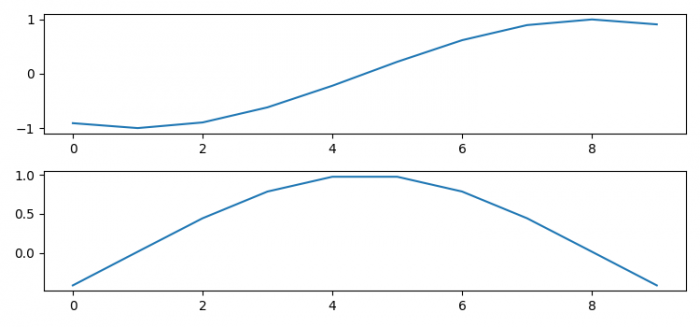# How to plot multiple graphs in Matplotlib?

To plot multiple graphs in matplotlib, we will use the following steps −

## Steps

• Create x, y1 and y2 data points using numpy.

• Add a subplot to the current figure at index 1.

• Plot curve 1 using and y1.

• Add a subplot to the current figure at index 2.

• Plot curve 2 using x and y2.

• To display the figure, use show() method.

## Example

import numpy as np
from matplotlib import pyplot as plt
plt.rcParams["figure.figsize"] = [7.50, 3.50]
plt.rcParams["figure.autolayout"] = True
x = np.linspace(-2, 2, 10)
y1 = np.sin(x)
y2 = np.cos(x)
plt.subplot(211)
plt.plot(y1)
plt.subplot(212)
plt.plot(y2)
plt.show()

## Output